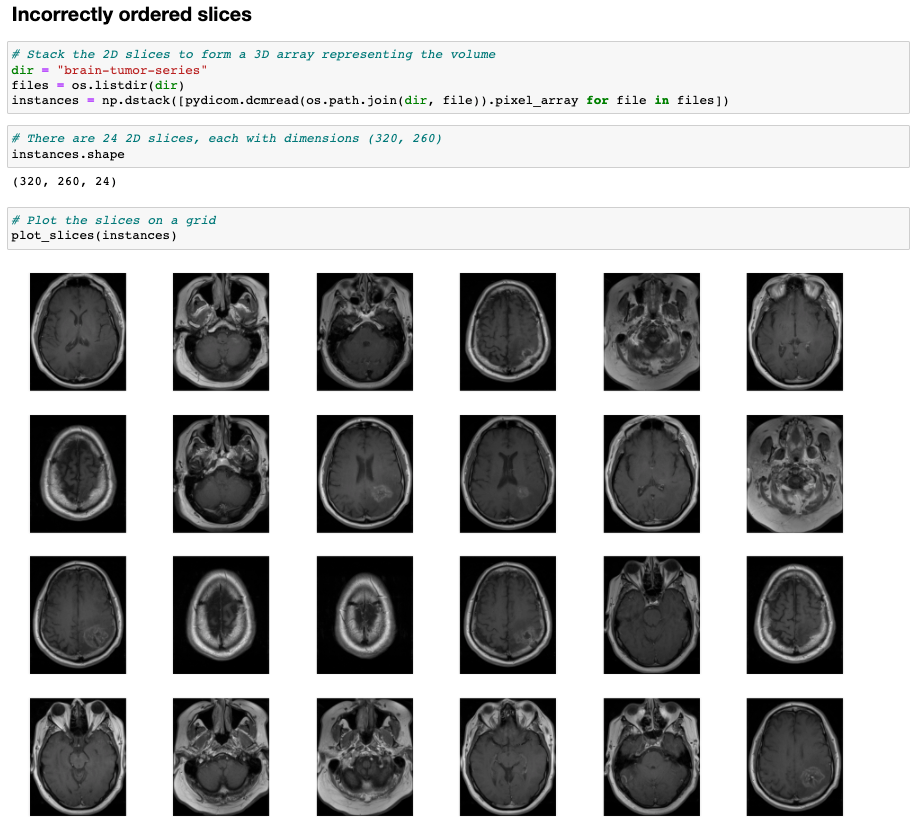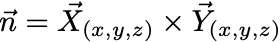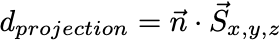# Introduction to DICOM Coordinate Systems

Shivam Sharma

January 15, 2022

Oops! Something went wrong while submitting the form.This blog aims to provide ML/CV engineers with a working knowledge of DICOM coordinate systems, and positioning and orientation of DICOM images.

If you’re completely new to DICOM, i’ve written an Introduction to DICOM data for computer vision engineers here.

## 3D Images

What is a 3D Image? A medical imaging device like a CT scanner takes images of a persons body from different angles to create a series of 2D cross-sectional images through an image reconstruction algorithm like back-projection.

Stacking these 2D slices together gives us a 3D image — see the GIF below that shows a CT scan of the chest.

Normal 2D images (like a picture of a dog) just contain information about the pixels in the image — 3D images are more complex, each 2D slice is stored in a file that contains pixel data information as well as information about the position and orientation of these 2D slices in 3D space.

## Why should we care about the position of 2D images in 3D space?

In this article, i’m going to explore two important and common functions performed with DICOM images, that require properly understanding the positioning and orientation of 2D slices in three dimensions / physical space.

• Correctly ordering 2D slices to form a 3D image.
• Representing voxels of an image in a physical dimension (mm) to do things like measure objects in images (e.g. tumors)

Let’s start by building some working knowledge of the coordinate frames DICOM relies on — Reference Coordinate System (RCS), and the Image Plane Module.

## DICOM Reference Coordinate System

The DICOM standard defines a Reference Coordinate System (RCS) that is patient oriented. The RCS will allow us to measure the position and orientation of an image with respect to the patient. The RCS is defined below.

• The positive X direction is towards the left of the patient.
• The positive Y direction is towards the back of the patient (anterior to posterior).
• The positive Z direction is towards top of the patient (inferior to superior).

## Image Plane Module

The Image Plane Module in the DICOM orients the 2D pixel array of each slice in three dimensions with respect to the patient.

The Image Plane Module defines multiple attributes that completely define the position/orientation of the image — the important ones are defined below Image Position Patient, Image Orientation Patient, Pixel Spacing, Slice Thickness.

### Image Position Patient (IPP)

The first important attribute is the IPP which gives the position of each slice in three dimensions with respect to the RCS — see figure above.

The IPP defines the x, y, and z coordinates of the upper left hand corner (center of the first voxel transmitted) of the image, in mm (measured from the RCS).

### Image Orientation Patient (IOP)

In addition to knowing where the 2D slice is in 3D, we also need to know how it is rotated in 3D space. This information is provided in the IOP — see figure above.

The direction cosines of the first row and the first column with respect to the patient.

Note — the orientation of the third axis is not explicitly communicated, but can be inferred by taking a cross-product of the first two direction cosines.

In the DICOM standard, a image coordinate frame is defined as follows:

• The positive row axis is left to right, or from the first pixel transmitted of a row to the last.
• The positive column axis is top to bottom, or from the first pixel of a column to the last pixel in that column.
• A cross product between the row axis and column axis, will give us the axis along which the image is stacked.

### Pixel Spacing

When the image is stored as Pixel Data in an array, the distance between each voxel (along row and column) is 1 pixel. However, in physical space, this distance can be different — the true physical spacing between pixels is given by Pixel Spacing (see the figure above).

Physical distance in the patient between the center of each pixel, specified by a numeric pair — adjacent row spacing (delimiter) adjacent column spacing in mm.

### Slice Thickness

Okay, so from the Pixel Spacing element we know how voxels are spaced in the plane of the image — but what about in the axis normal to the image plane? Slice thickness tells us the ‘height’ of a voxel in mm (see the figure above).

Nominal slice thickness, in mm.

Note — slice thickness is an element of Type 2 i.e. it is required in DICOM files, but its value can be 0. If it is 0, the slice thickness needs to be inferred from the IPP.

### Position of a DICOM voxel, in three dimensional space

Now that we’ve gone over some of the core concepts, let’s revisit one of our topics from earlier — representing voxels of an image in 3D physical space.

Looking at the image above, if we wanted to know the position of pixel (i,j) within the RCS in (x,y,z) millimeters, we can apply a coordinate transform.

Let’s try and work that out — we can use the values we’ve discussed so far to construct the following equation for the X position of the pixel w.r.t RCS.

If we do this for all components — x,y,z, we get the following transformation which gives us the position of all the pixels in a DICOM image in physical space.

P_xyz: The coordinates of the voxel (i,j) in mm w.r.t RCS (mm).

S_xyz: The Image Position Patient, which gives us position of the center of the first voxel w.r.t RCS (mm).

X_xyz, Y_xyz: The row and column direction cosines of the Image Orientation Patient (unit vectors).

i, j: Column index and row index respectively (index).

∆i, ∆j: Row and Column pixel spacing values (mm).

Using this transformation matrix, we can find the position of all voxels in 3D space, and can make measurements/alignments in the image with real physical units like millimeters.

## Sorting 2D slices of a 3D image in correct order

Finally, let’s revisit the second key topic we discussed earlier correctly ordering 2D slices to form a 3D image.

Let’s start by seeing what happens if you don’t correctly order your slices. If we simply read in the 2D slices and visualize the images, we’ll see the grid in the image below has randomly positioned slices — this is problematic if you’re trying to get an intuitive view of your 3D image.(Left) Instances of a CT scan, (Right) incorrectly ordered slices visualized.

To correctly order these slices, we need to order them in the direction of the imaging axis (axis normal to the image plane). If we knew these images were taken along the RCS X axis, we could easily order the images by using the IPP X values. However, nothing from the DICOM files tells us what the imaging axis is, so we have to infer it.

Referring back to our definition of IOP, we know we can find the imaging axis by performing a cross product of the row and column IOP vectors:The normal vector is the imaging axis, i.e. the axis along which the slices stack.

Now, we want to find the projection of the IPP for each slice along this normal vector — this projection will give as a scalar value which tell us the position of each 2D slice along the imaging axis.

To find this projection, we need to take the dot product. We can then order the slices using this projection.The projection gives us the ‘distance’ of each slice along the normal imaging axis.

Now let’s do this in code. Compute the projection of the IPP on the normal vector for all the slices.

Sort the slices based on the projection and visualize.

There you go — perfectly ordered slices, and a perfect understanding of the position and orientation of DICOM images!!

## References

1. DICOM Standard — https://www.dicomstandard.org/current.
2. UCL, DICOM for MRI — http://www.cs.ucl.ac.uk/fileadmin/cmic/Documents/DavidAtkinson/DICOM.pdf.